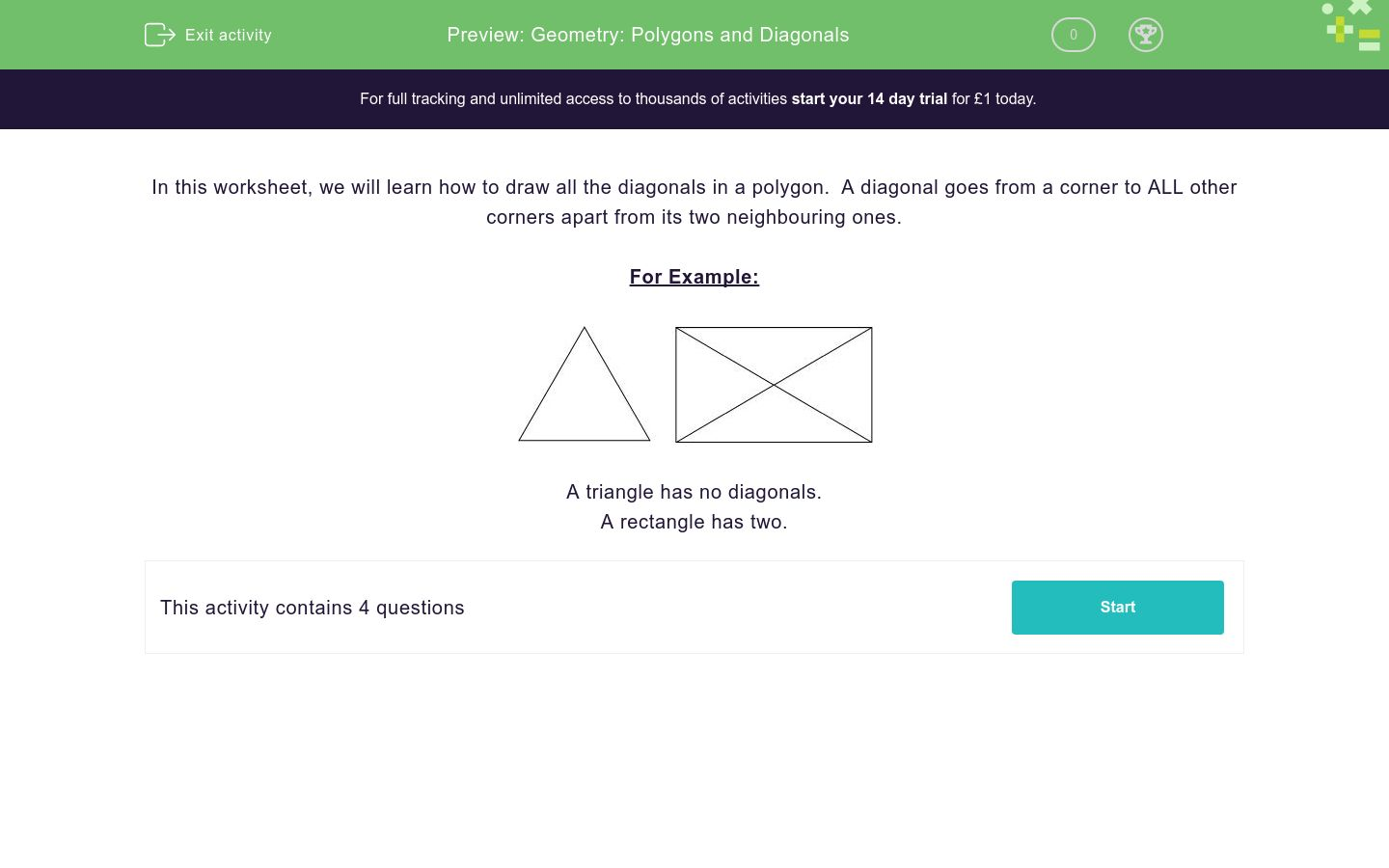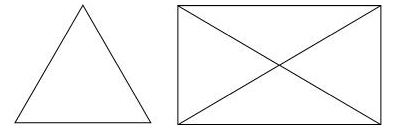# Geometry: Polygons and Diagonals

In this worksheet, students learn about diagonals in polygons.Key stage:  KS 2

Curriculum topic:   Geometry: Properties of Shapes

Curriculum subtopic:   Understand Polygons

Difficulty level:### QUESTION 1 of 10

In this worksheet, we will learn how to draw all the diagonals in a polygon.  A diagonal goes from a corner to ALL other corners apart from its two neighbouring ones.

For Example:A triangle has no diagonals.

A rectangle has two.

Write down the number of diagonals in the following shape.Write down the number of diagonals in the following shape.Write down the number of diagonals in the following shape.Write down the number of diagonals in the following shape.• Question 1

Write down the number of diagonals in the following shape.4
EDDIE SAYS
There should be 2 diagonals coming out from each corner. But don't count any twice!
• Question 2

Write down the number of diagonals in the following shape.6
EDDIE SAYS
There are 6 diagonals in this shape. Try drawing it out on paper.
• Question 3

Write down the number of diagonals in the following shape.14
• Question 4

Write down the number of diagonals in the following shape.